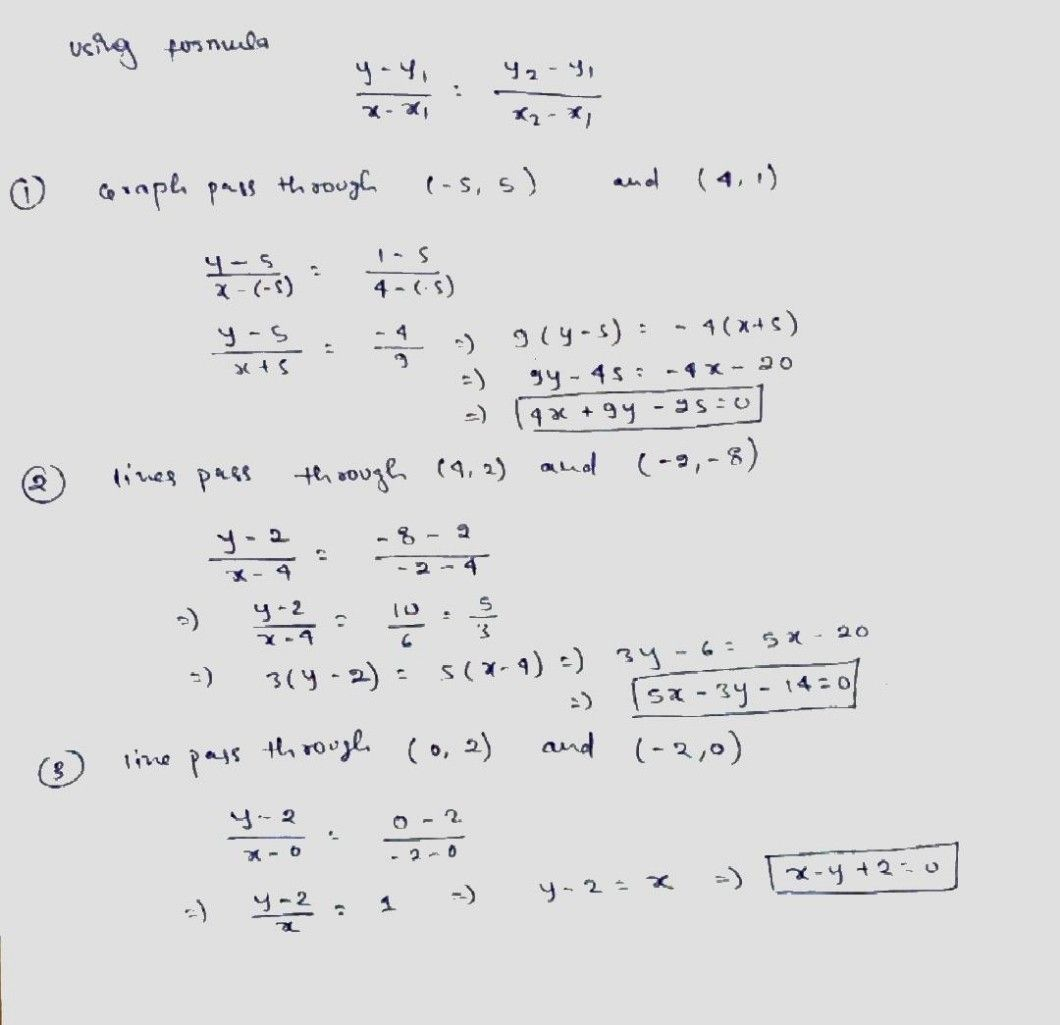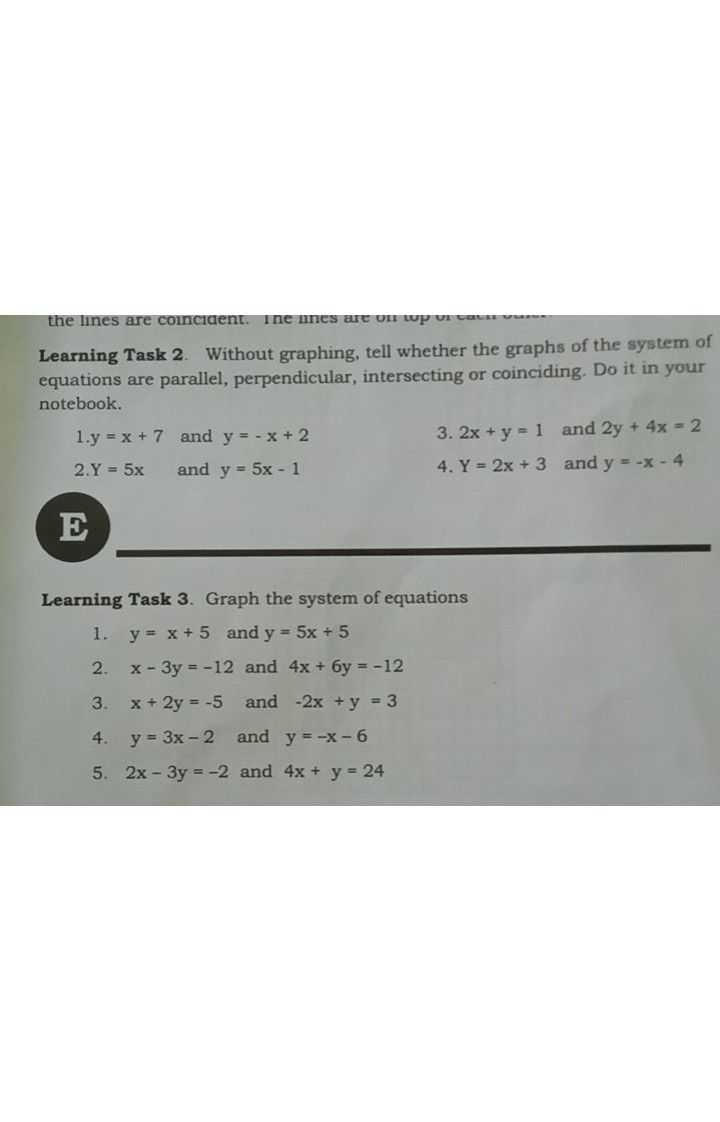Symbol
ProblemLearning Task $4:$ Do the following in your notebook. $A.$ Find the equation of the line given the graph. $1$ $2$ $3.$ $3$ $4$ $2$ $\bar{2}$ $9$ $5$ $3$ $b$ $2$ $2$ $2$ $3$
7th-9th grade
Other
SolutionQanda teacher - AskShivaniIf you have any doubt feel free to ask:) Dear, Please evaluate the answer and give gift coins(it does not reduce your coin balance.) for giving gift coins Click on evaluate. Then press the + icon 3 times(3000).
Please evaluate:- 1. Click on top right corner EVALUATE button. 2.Select 5 star. 3.Click on gift coins + three times(C3000)(Not reduce your coins). 4.Click on DONE button on top right corner.Student